Circle CuttingDetermining the maximum number of pieces in which it is possible to divide a Circle for a given number of cuts is called the circle cutting, or sometimes Pancake Cutting, problem. The minimum number is always, whereis the number of cuts, and it is always possible to obtain any number of pieces between the minimum and maximum. The first cut creates 2 regions, and theth cut createsnew regions, so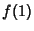(1)(2)(3)

Therefore,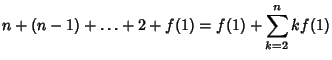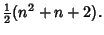(4)

Evaluating for, 2, ... gives 2, 4, 7, 11, 16, 22, ... (Sloane's A000124).A related problem, sometimes called Moser's Circle Problem, is to find the number of pieces into which a Circle is divided ifpoints on its Circumference are joined by Chords with no three Concurrent. The answer is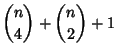(5)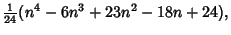(6)

(Yaglom and Yaglom 1987, Guy 1988, Conway and Guy 1996, Noy 1996), whereis a Binomial Coefficient. The first few values are 1, 2, 4, 8, 16, 31, 57, 99, 163, 256, ... (Sloane's A000127). This sequence and problem are an example of the danger in making assumptions based on limited trials. While the series starts off like, it begins differing from this Geometric Series at.

See also Cake Cutting, Cylinder Cutting, Ham Sandwich Theorem, Pancake Theorem, Pizza Theorem, Square Cutting, Torus Cutting

References

Conway, J. H. and Guy, R. K. How Many Regions.'' In The Book of Numbers. New York: Springer-Verlag, pp. 76-79, 1996.

Guy, R. K. The Strong Law of Small Numbers.'' Amer. Math. Monthly 95, 697-712, 1988.

Noy, M. A Short Solution of a Problem in Combinatorial Geometry.'' Math. Mag. 69, 52-53, 1996.

Sloane, N. J. A. Sequences A000124/M1041 and A000127/M1119 in An On-Line Version of the Encyclopedia of Integer Sequences.'' http://www.research.att.com/~njas/sequences/eisonline.html and Sloane, N. J. A. and Plouffe, S. The Encyclopedia of Integer Sequences. San Diego: Academic Press, 1995.

Yaglom, A. M. and Yaglom, I. M. Problem 47. Challenging Mathematical Problems with Elementary Solutions, Vol. 1. New York: Dover, 1987.